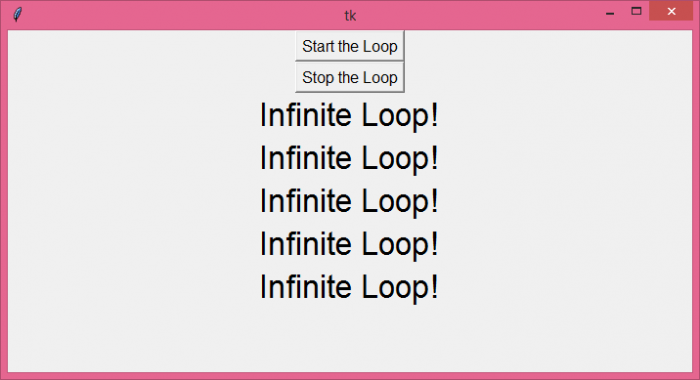# How to run an infinite loop in Tkinter?

To run an infinite loop in Tkinter, we will use the after method to call a method recursively after a specified time period until the user decides to stop the loop. Let's take a simple example and see how to start and stop an infinite loop.

## Steps −

• Import the required libraries and create an instance of tkinter frame.

• Set the size of the frame using win.geometry method.

• Next, create a user-defined function "infinite_loop" which will call itself recursively and print a statement on the window.

• Define two more user-defined functions, start() and stop(), to control the infinite_loop. Define a global variable "condition". Inside start(), set condition=True and inside stop(), set condition=False.

• Create two buttons to call the start() and stop() functions.

• Use the after() method to call the infinite_loop recursively after every 1 second.

• Finally, run the mainloop of the application window.

## Example

# Import the required library
from tkinter import *

# Create an instance of tkinter frame
win=Tk()

# Set the size of the Tkinter window
win.geometry("700x350")

# Define a function to print something inside infinite loop
condition=True
def infinite_loop():
if condition:
Label(win, text="Infinite Loop!", font="Arial, 25").pack()

# Call the infinite_loop() again after 1 sec win.after(1000, infinite_loop)

def start():
global condition
condition=True

def stop():
global condition
condition=False

# Create a button to start the infinite loop
start = Button(win, text= "Start the Loop", font="Arial, 12", command=start).pack()
stop = Button(win, text="Stop the Loop", font="Arial, 12", command=stop).pack()

# Call the infinite_loop function after 1 sec.
win.after(1000, infinite_loop)

win.mainloop()

## Output

When you run this code, it will produce the following output −Click the button "Start the Loop" to run the infinite loop which will keep printing "Infinite Loop!" after every second. Click "Stop the Loop" to stop the infinite loop.